Fall 2003

# Mathematics Math21b Fall 2003

## Linear Algebra and Differential Equations

Office: SciCtr 434
Email: knill@math.harvard.edu# Fourier Series

(Coordinates in infinite dimensions)
For a periodic function f, the Fourier coefficients are

 a0 = (f,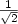), an = (f, cos(n x) ), bn = (f,sin(n x))

where (f,g) =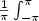f(x) g(x) dx is the dot product. The function f can be recovered as a real Fourier series

 f(x) = a0+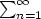an cos(n x) + bn sin(n x)

The functions, cos(nx), sin(n x) form an orthonormal set of functions on the space of periodic functions (*). The Fourier coefficients are the coordinates of f in that basis.
Using the orthonormality relations, we get the Perseval equality

 ||f||2 = a02 +(an2 + bn2)

where ||f||=(f,f).
The complex Fourier coefficients are

 cn = (f,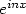),

where (f,g) =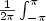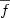(x) g(x) dx is the dot product. (Note the additional factor 2 in the denominator). The function f can be recovered as a complex Fourier series

 f(x) =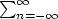cnThe functions form an orthonormal set of functions on the space of complex valued periodic functions. The Fourier coefficients are the coordinates of f in that basis.
Using the orthonormality relations, we get the Perseval equality

 ||f||2 =|cn|2

where ||f||=(f,f).

 (*) To see orthonormality, the trigonometric identities are useful: ``` 2 cos(nx) cos(my) = cos(nx-my) + cos(nx+my) 2 sin(nx) sin(my) = cos(nx-my) - cos(nx+my) 2 sin(nx) cos(my) = sin(nx+my) + sin(nx-my) ``` are useful as well as to know that integrating sin(n x) or cos(n x) over [0,2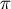] is zero for all n different from 0.

## Examples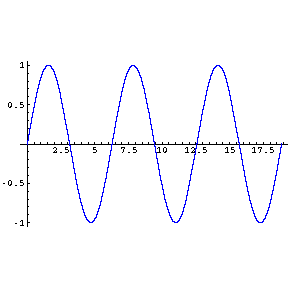f(x) = sin(x)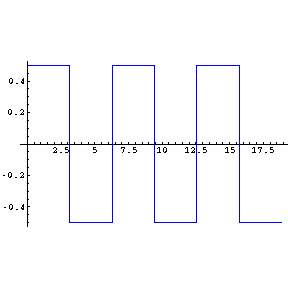f(x) = sign(sin(x))/2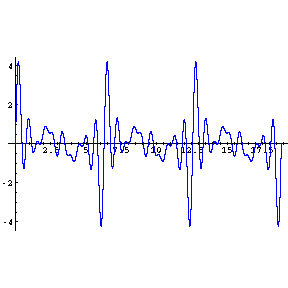f(x) = trigonometric polynomial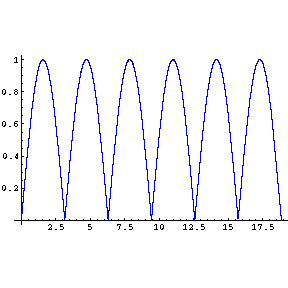f(x) = |sin(x)|

## Mathematica Source

 The pictures and sounds in the above 4 examples were generated with the following Mathematica procedure:

 ```(* Oliver Knill, 12/7/2003 Math21b Fall 2003 *) (* Mathematica procedures illustrating sound synthesis *) PlaySong[hull_,tune_,name_,ground_]:=Module[{}, u=ToCharacterCode[name]; imagefilename=FromCharacterCode[Join[u,{46,103,105,102}]]; soundfilename=FromCharacterCode[Join[u,{46,119,97,118}]]; scale[n_]:=ground*2^(n/12); beatlength=1/5; songlength=Length[tune]*beatlength; frequency[x_]:=tune[[1+Floor[x/beatlength]]]; song[t_]:=hull[scale[frequency[t]]*t]; P=Play[song[t],{t,0,songlength}]; S=Plot[hull[x],{x,0,6Pi}]; Export[soundfilename,P,"WAV"]; Display[imagefilename,S,"GIF"]; ] t1={0,0,4,4,7,7,5,4,2,2,0,4,7,4,0,0,0,0,0,2,0}; f1[x_]:=Sin[x]; n1="sin"; g1=2000; PlaySong[f1,t1,n1,g1] t2={0,0,0,0,4,4,4,4,2,4,2,0,0,4,6,7,7,6,7,6,3}; f2[x_]:=Sign[Sin[x]]; n2="heavy"; g2=3000; PlaySong[f2,t2,n2,g2] t3={0,0,0,0,4,4,4,4,2,4,2,0,0,4,6,7,7,6,7,6,3}; f3[x_]:=Abs[Sin[x]]; n3="saw"; g3=1500; PlaySong[f3,t3,n3,g3] r={0.451808, 0.075485, 0.486453, 0.513869, 0.175862, 0.666061, 0.66505, 0.898679, 0.649515, 0.537377} f4[x_]:=Sum[Sin[k*x]*r[[k]],{k,Length[r]}] t4={1,1,1,8,8,8,3,4,3,1,1,1,8,8,11,13,13,11,8,9,6,8,8,8,8}; n4="sax"; g4=2000; PlaySong[f4,t4,n4,g4] ```
All functions are 2periodic, defined on [-,] and then periodically continued.

 f(x) = cos(2x) + 3 sin(17 x) + 5 A trigonometric polynomial is already a Fourier series f(x) = x f(x) =(2 (-1)n+1/n) sin(n x) f(x) = |x| f(x) =/2 -4/((2n-1)2) sin((2n-1) x)

## Approximation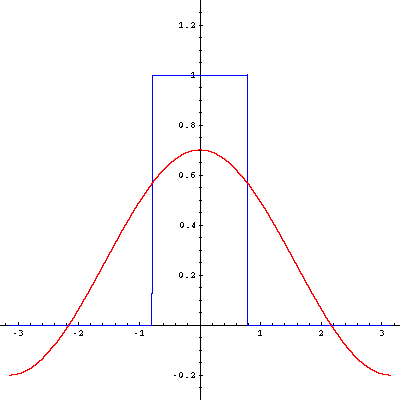The animation shows the first 60 Fourier approximations of the Heavyside function f(x)=sign(cos(x)). Because this function is discontinuous, the approximation is not so fast. Near the discontinuity, one can observe the Gibbs phenomenon, an overshoot near the discontinuity which while getting more and more narrow, does not go to zero in amplitude. Fourier coefficients can be computed very easily in Mathematica for any function f:

 ```a0[f_]:=NIntegrate[f[x]/Sqrt,{x,0,2Pi}]/Pi; a[f_,n_]:=NIntegrate[f[x]*Cos[n*x],{x,0,2Pi}]/Pi; b[f_,n_]:=NIntegrate[f[x]*Sin[n*x],{x,0,2Pi}]/Pi; ```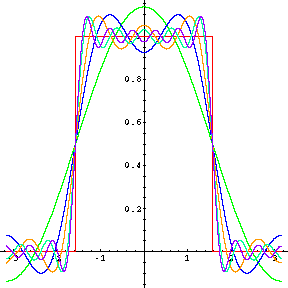## Fourier series in 2DEach of the three color channels of a picture can be realized as a function f(x,y) of two variables. These three functions red(x,y), green(x,y), blue(x,y) determine the picture. The Fourier coefficients of functions of two variables are defined similarly as in one dimension. The one dimensional integral becomes a double integral. As a basis, one can take fn,m = exp(i n x) exp(i m y) as well as their real analogues. The animation shows the first 70 Fourier approximations to a picture of dimensions 150 times 150 pixels. (While this could be done easily in Mathematica also, the animation here has been produced with a little standalone C program.) You can also observe the Gibbs phenomenon here. The JPG compression standard uses discrete cosine transform on blocks of 8 x 8 pixels.
Back to the main page